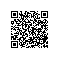# SLS机器学习最佳实战：日志聚类+异常告警

## 1.手中的锤子都有啥？

• 上下文查询
• 实时Tail和智能聚类，以提高问题调查效率
• 提供多种时序数据的异常检测和预测函数，来做更智能的检查和预测
• 数据分析的结果可视化
• 强大的告警设置和通知，通过调用webhook进行关联行动## 2.平台实验

### 2.1 实验数据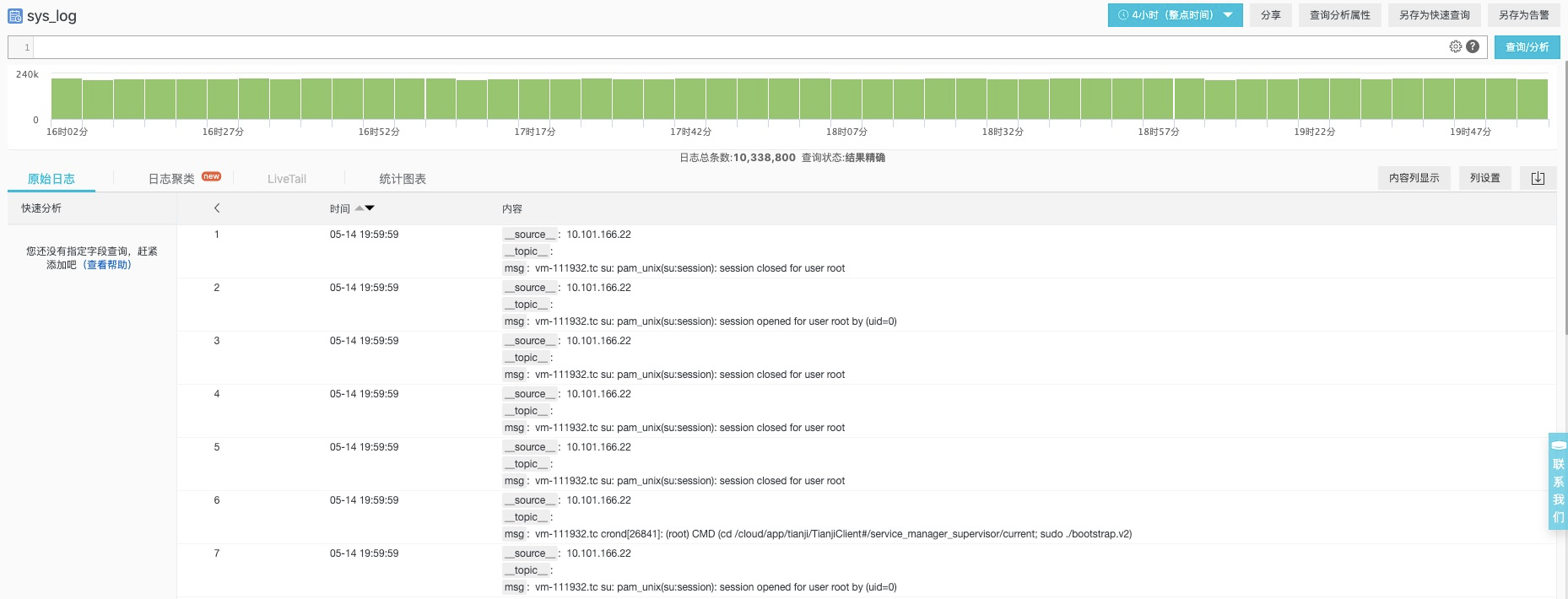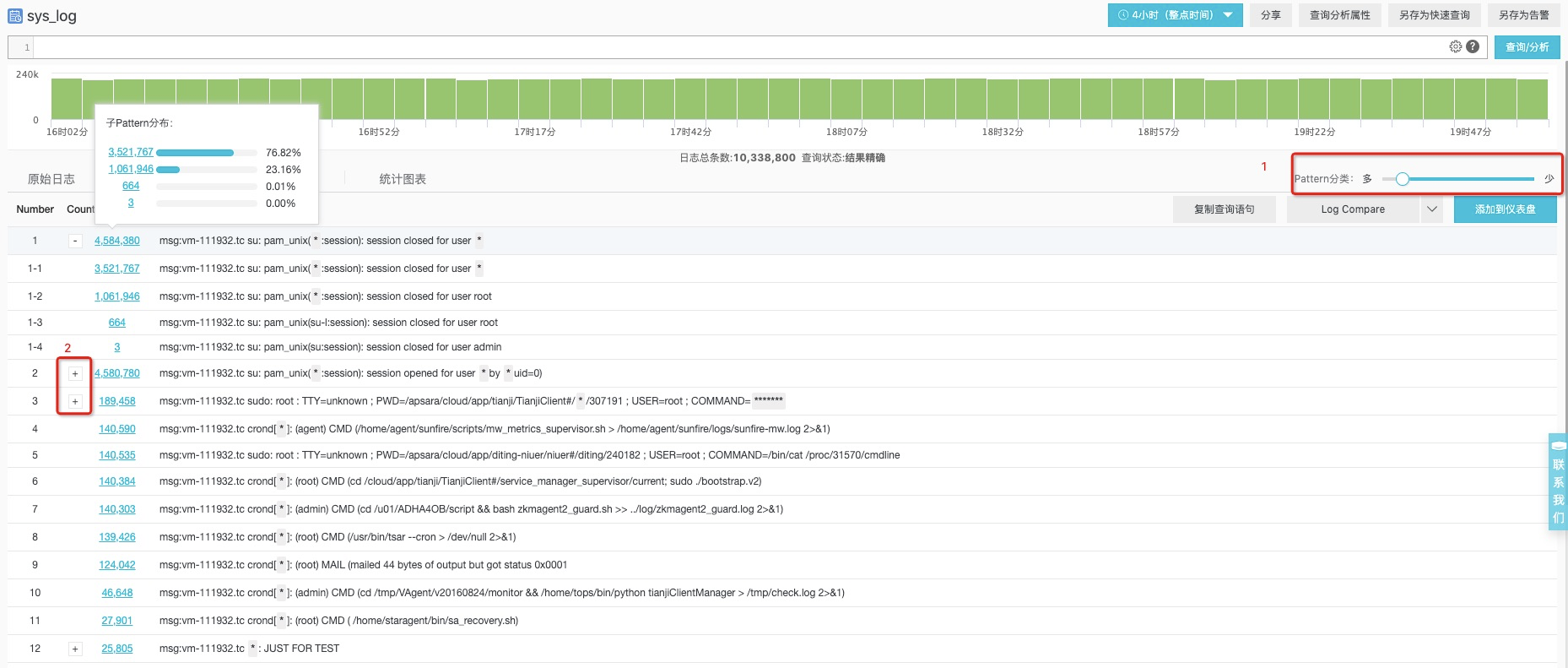### 2.2 生成子模式的时序信息

msg:vm-111932.tc su: pam_unix(*:session): session closed for user root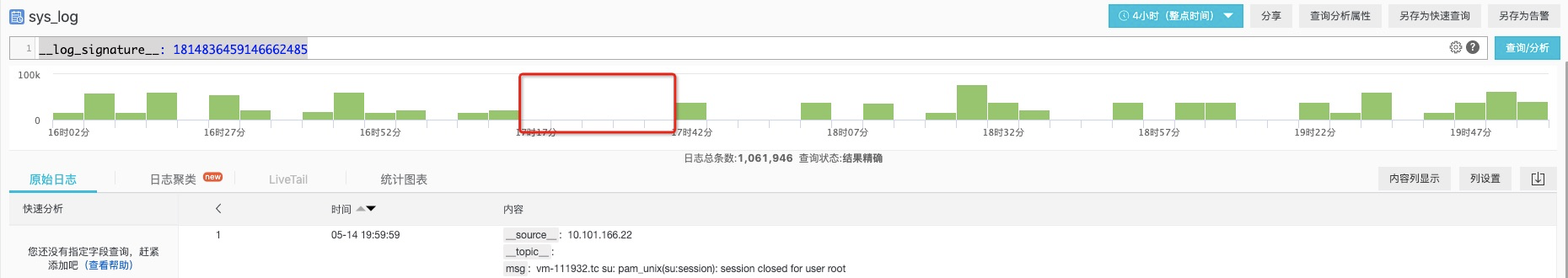__log_signature__: 1814836459146662485 |
select
date_trunc('minute', __time__) as time,
COUNT(*) as num
from log GROUP BY time order by time ASC limit 10000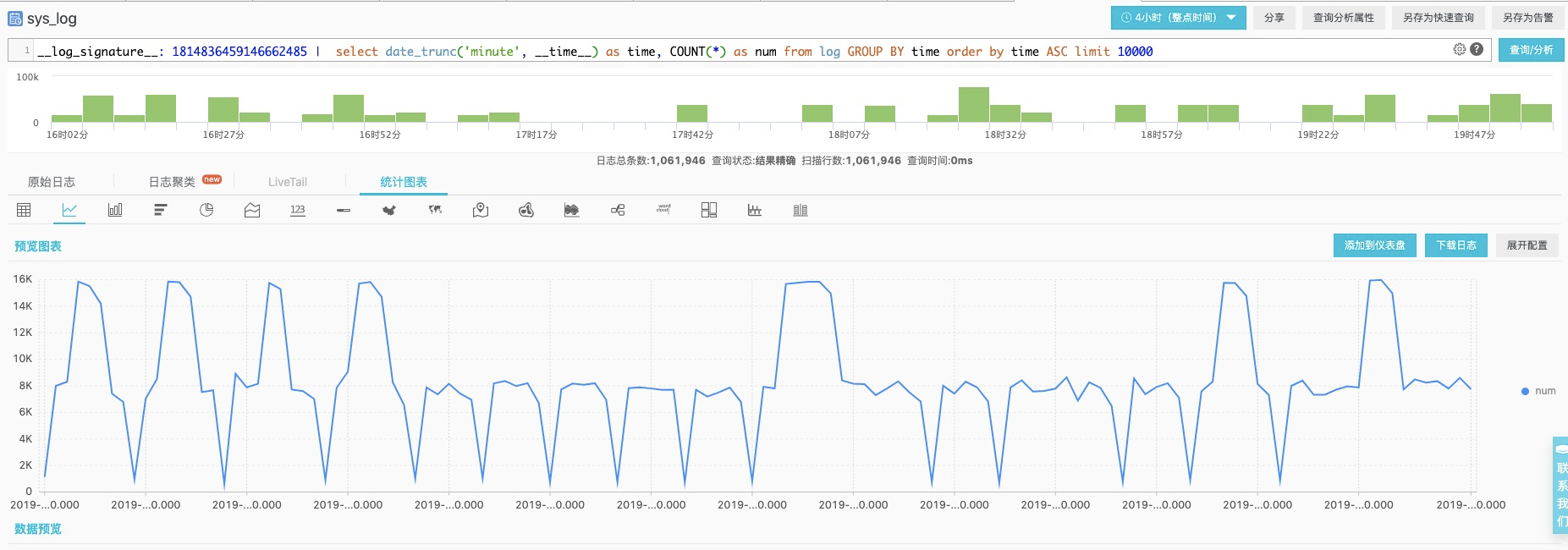__log_signature__: 1814836459146662485 |
select
time_series(time, '1m', '%Y-%m-%d %H:%i:%s', '0') as time,
avg(num) as num
from  (
select
__time__ - __time__ % 60 as time,
COUNT(*) as num
from log GROUP BY time order by time desc )
GROUP by time order by time ASC limit 10000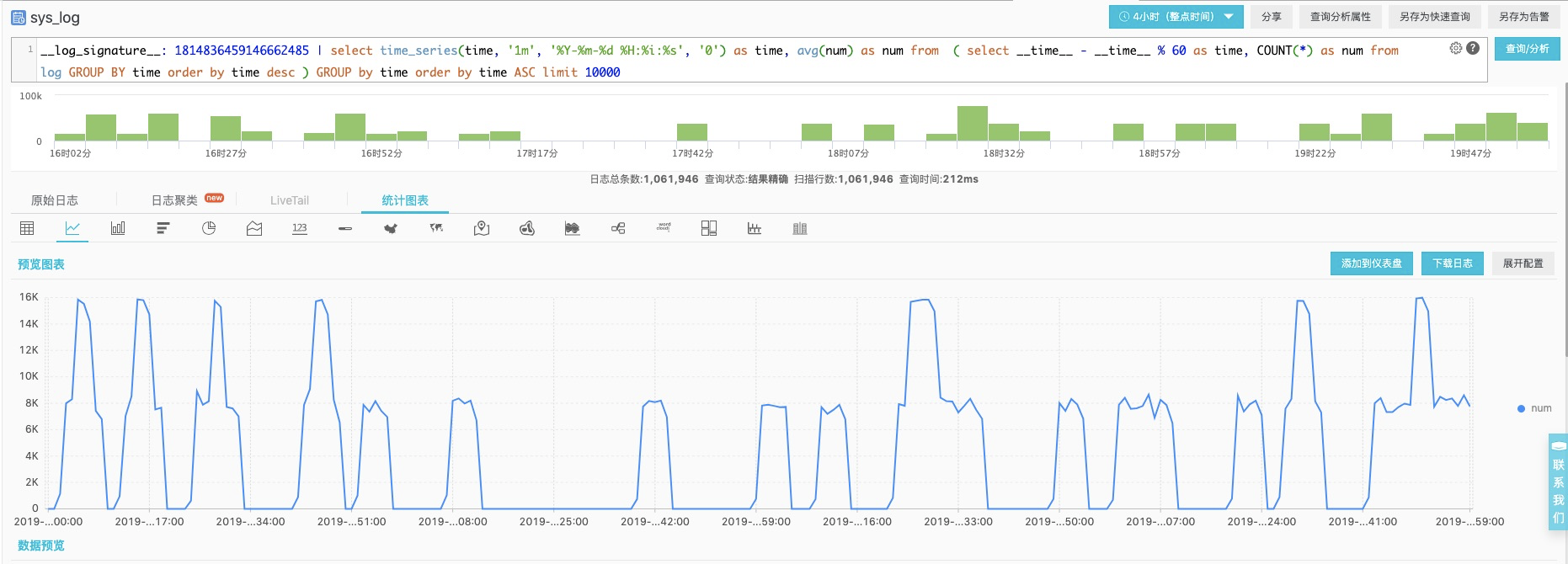### 2.3 对时序进行异常检测

__log_signature__: 1814836459146662485 |
select
ts_predicate_arma(to_unixtime(time), num, 5, 1, 1, 1, 'avg')
from  (
select
time_series(time, '1m', '%Y-%m-%d %H:%i:%s', '0') as time,
avg(num) as num
from  (
select
__time__ - __time__ % 60 as time,
COUNT(*) as num
from log GROUP BY time order by time desc )
GROUP by time order by time ASC ) limit 10000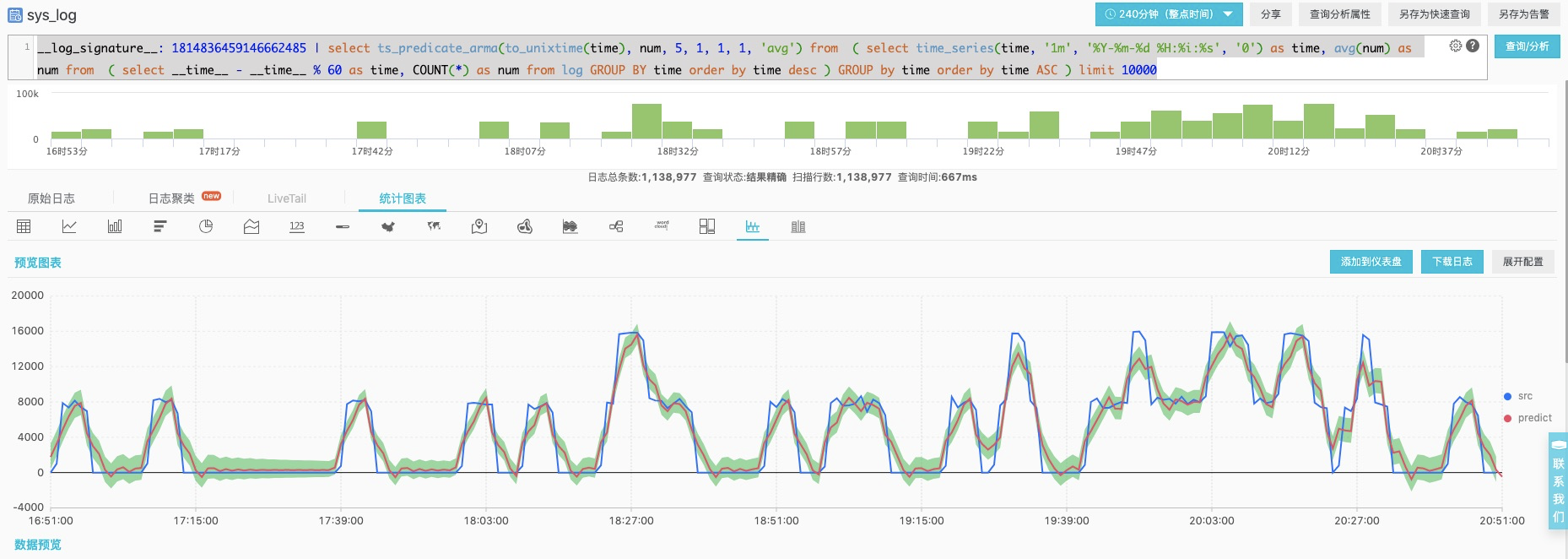### 2.4 告警该如何设置

• 将机器学习函数的结果拆解开
__log_signature__: 1814836459146662485 |
select
t1 as unixtime, t1 as src, t1 as pred, t1 as up, t1 as lower, t1 as prob
from  (
select
ts_predicate_arma(to_unixtime(time), num, 5, 1, 1, 1, 'avg') as res
from  (
select
time_series(time, '1m', '%Y-%m-%d %H:%i:%s', '0') as time,
avg(num) as num
from  (
select
__time__ - __time__ % 60 as time,
COUNT(*) as num
from log GROUP BY time order by time desc )
GROUP by time order by time ASC )) , unnest(res) as t(t1)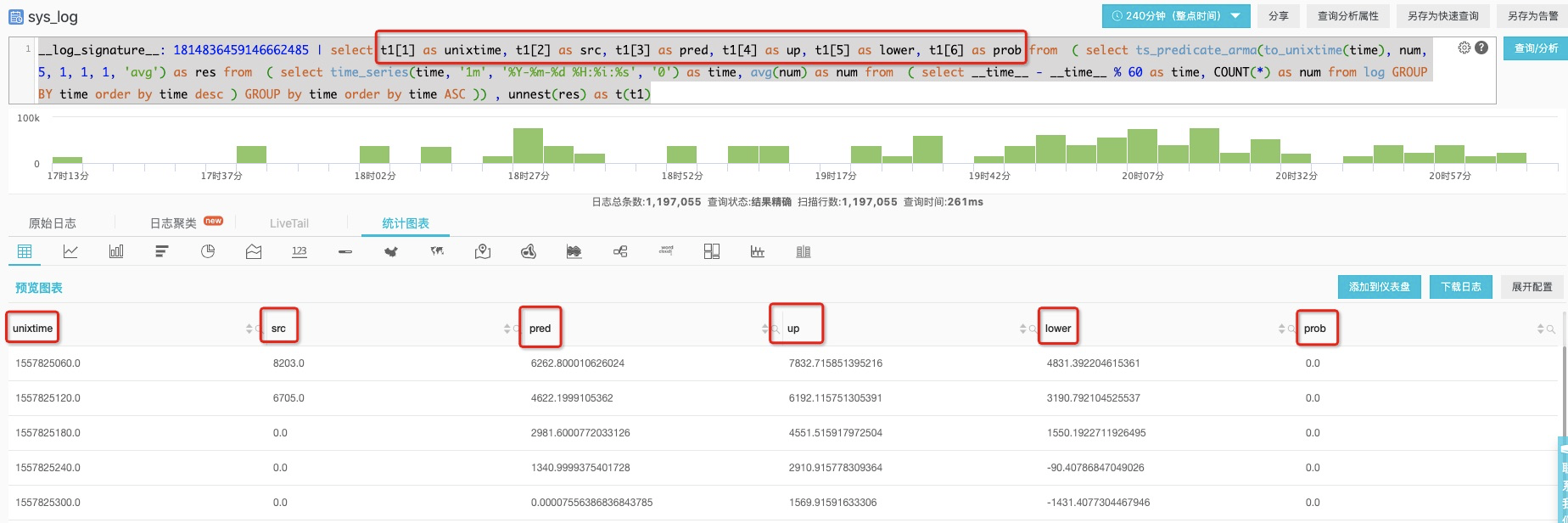• 针对最近两分钟的结果进行告警
__log_signature__: 1814836459146662485 |
select
unixtime, src, pred, up, lower, prob
from  (
select
t1 as unixtime, t1 as src, t1 as pred, t1 as up, t1 as lower, t1 as prob
from  (
select
ts_predicate_arma(to_unixtime(time), num, 5, 1, 1, 1, 'avg') as res
from  (
select
time_series(time, '1m', '%Y-%m-%d %H:%i:%s', '0') as time,
avg(num) as num
from  (
select
__time__ - __time__ % 60 as time, COUNT(*) as num
from log GROUP BY time order by time desc )
GROUP by time order by time ASC )) , unnest(res) as t(t1) )
where is_nan(src) = false order by unixtime desc limit 2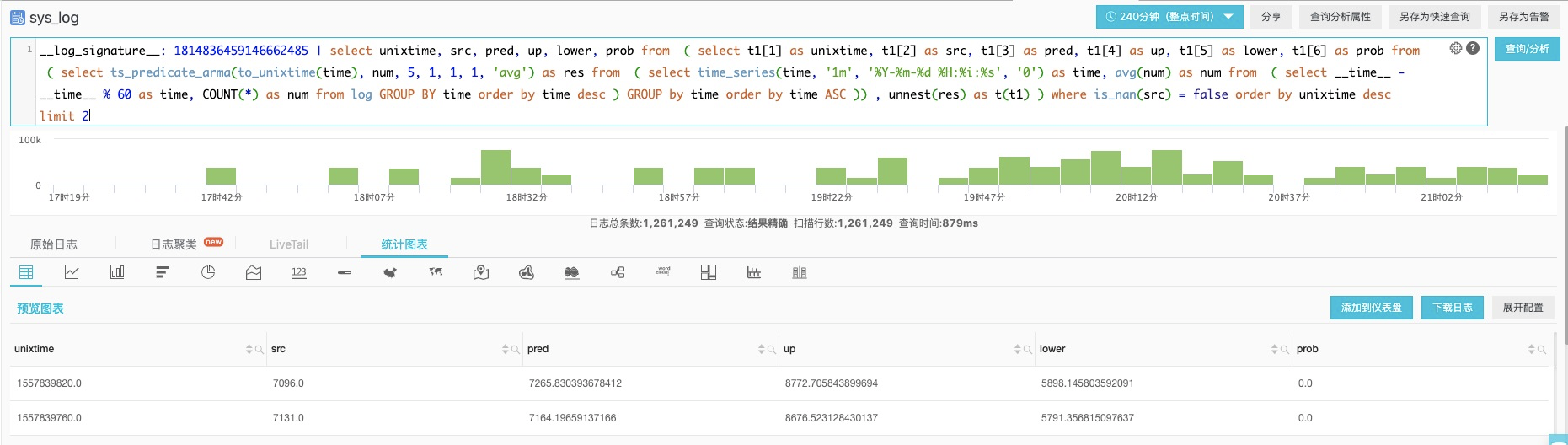• 针对上升点进行告警，并设置兜底策略
__log_signature__: 1814836459146662485 |
select
sum(prob) as sumProb, max(src) as srcMax, max(up) as upMax
from (
select
unixtime, src, pred, up, lower, prob
from  (
select
t1 as unixtime, t1 as src, t1 as pred, t1 as up, t1 as lower, t1 as prob
from  (
select
ts_predicate_arma(to_unixtime(time), num, 5, 1, 1, 1, 'avg') as res
from  (
select
time_series(time, '1m', '%Y-%m-%d %H:%i:%s', '0') as time, avg(num) as num
from  (
select
__time__ - __time__ % 60 as time, COUNT(*) as num
from log GROUP BY time order by time desc )
GROUP by time order by time ASC )) , unnest(res) as t(t1) )
where is_nan(src) = false order by unixtime desc limit 2 )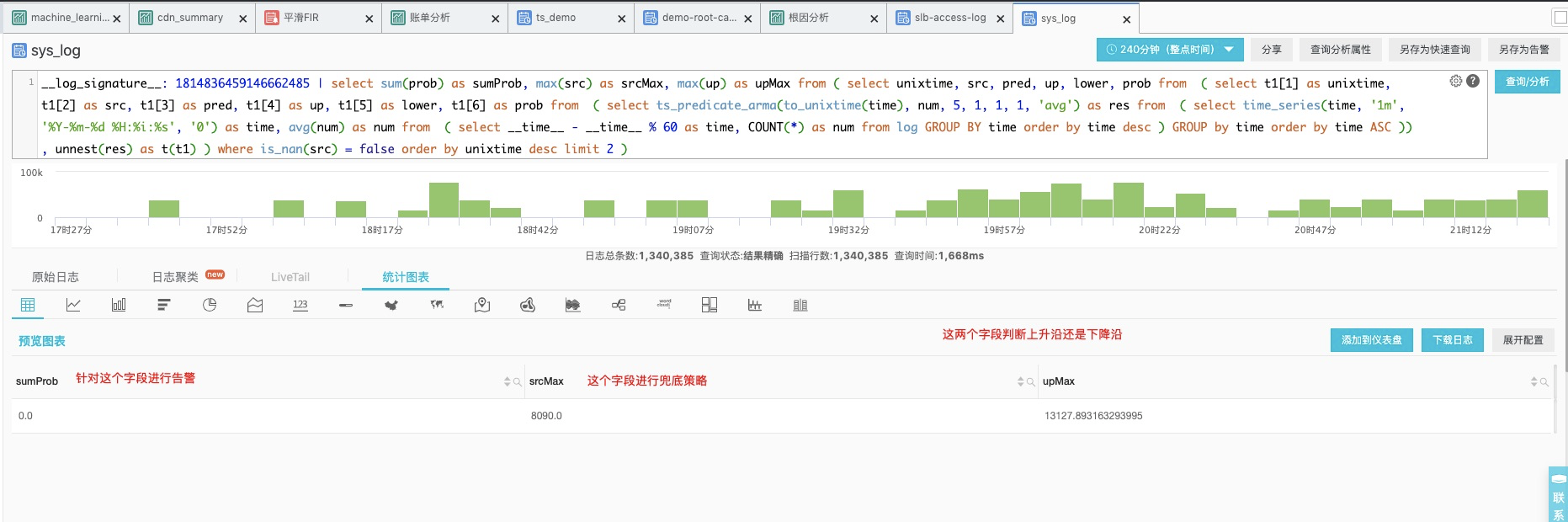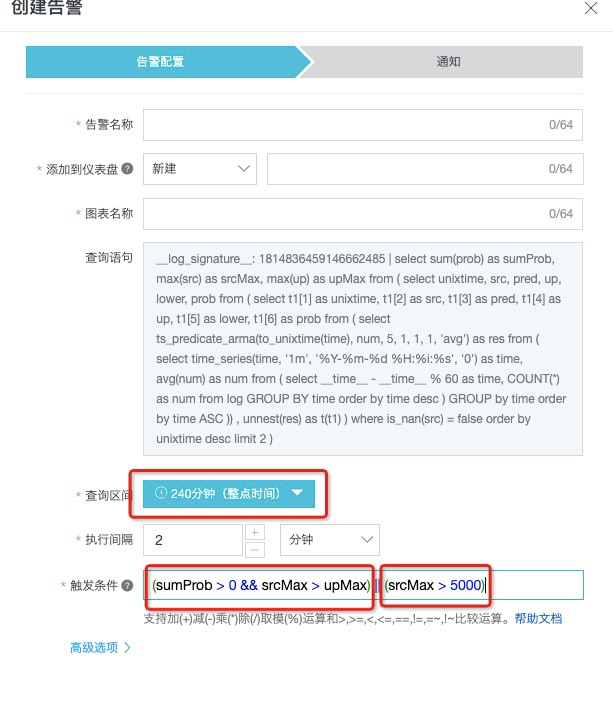## 3.硬广时间

### 3.1 日志进阶### 3.2 联系我们